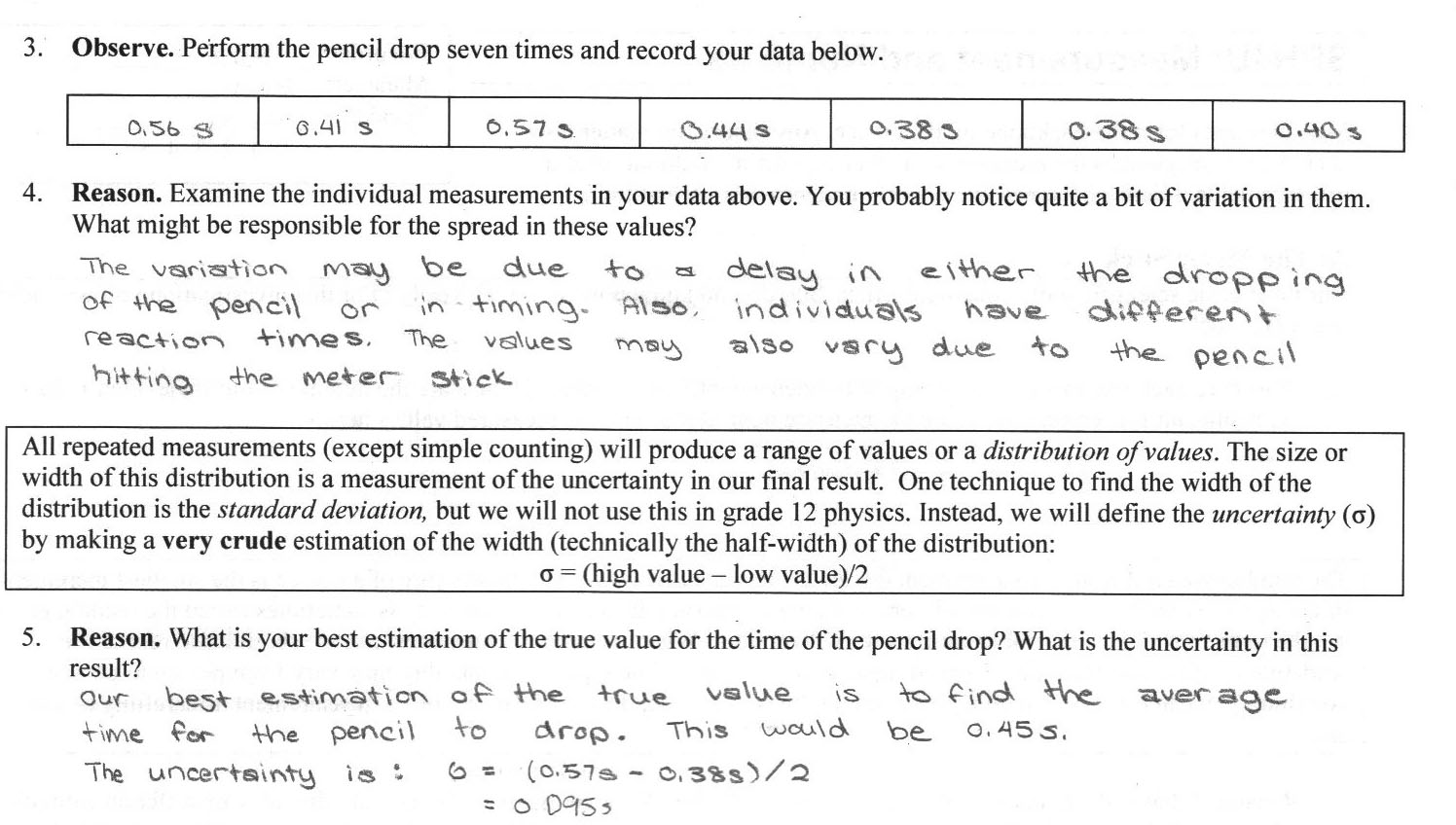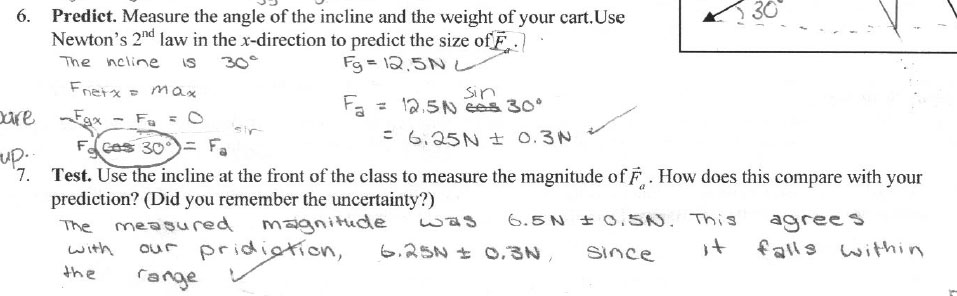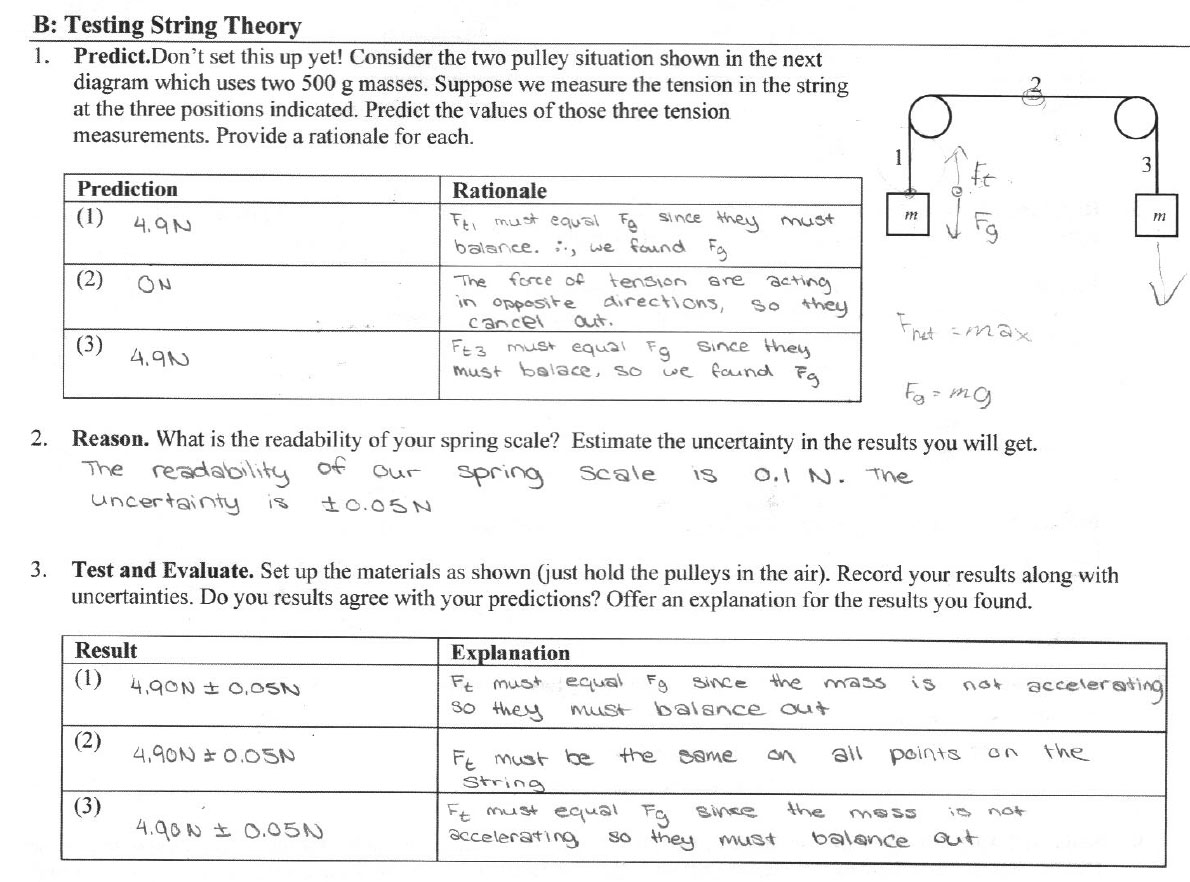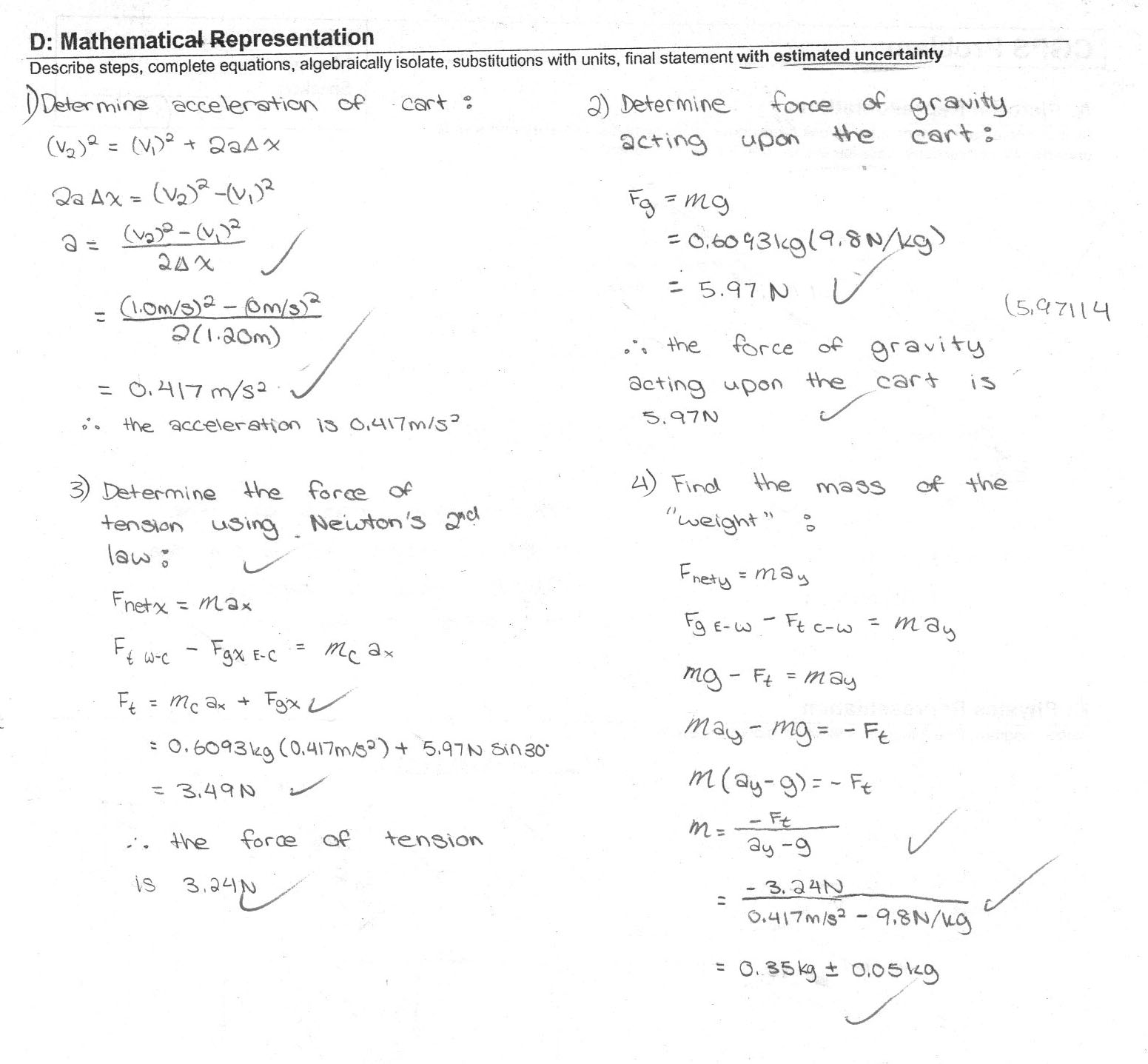.boxed { border: 1px solid green ; }

# A Numbers Game: the Significant Digits Racket

Chris Meyer, OAPT VP teaching and learning, Assistant curriculum leader York Mills C. I.
Christopher.meyer@tdsb.on.ca

OK, let’s begin by admitting that we are all playing a numbers game. Or, at least, we make our students play this game where they bet their marks on correctly figuring out the last digit to write down in their answers. (The classic numbers game is an illegal betting pool where people try to guess the last few digits of some “random” number like a stock price listing.) To make it sporting, we teach our students rules for identifying the significant digits in a given number and rules for deciding how many digits to keep after a calculation. Now, you likely know what happens next. For the rest of the year we are plagued by noisome questions during lessons and tests: “How many significant digits does this have?” “Is this two or three?” “Mr. Meyer, you started with 1000 and your final answer was 17.5 m/s ...” Sound familiar?

### I couldn’t handle the truth

I remember when I started my undergraduate physics training being perplexed by the mysterious absence of my cherished significant digits. No one (meaning the profs and TAs) was talking about them. I should have realized at the time what this meant, but I was just confused. I was wrestling with error analysis and standard deviations and didn’t put it all together: the rules I learned about significant digits in high school were bogus. Make believe. They played no role in good scientific thinking. It took me many years as a teacher to wise up to this and a few more to figure out what to do about it. To begin, let’s think about why the digits appearing in a number are important.

### The confidence game

Significant digits (like our significant others) have meaning in our lives. They tell us something important about our world and, in a certain sense, can be relied upon. People have built careers around finding the next digit in a particularly interesting number. Significant digits in high school are typically used as a statement of uncertainty: a distance written as 93 m is often interpreted as meaning we are confident the distance is actually “somewhere between 92.7 m and 93.4 m”. So our numbers game is also a confidence game. But what determines which digits in a number are the significant ones? The traditional answer to this question is that the written form of the number itself makes this determination. This idea is embodied in various rules, like the one that informs us the number 2000 has one significant digit. Unfortunately this approach is very problematic and leads to poor, or non-existent, scientific thinking. The reality is that by looking at any single number representing a physical quantity (170 km, 348 N, 0.740 s), we cannot determine anything about its significant digits or decide on our confidence in it.

The proper origin of significant digits is in the measurement process. Suppose we measure the time it takes for a ball to drop from a stairwell and find an average value of 1.75 s. How should we interpret the meaning of this value? Its meaning comes from a comparison with the true value of this quantity: the result that a patient and omniscient being would arrive at using really expensive equipment and great lab technique. The trick is, we never get to know the true value (sigh, all that expensive equipment...) So in our comparison of “1.75 s” with the true value, we would say something like this: we are really sure that the digit “1” is reliable, meaning the probability of the true value having a different digit in the ones decimal place is very small (maybe to a 5σ confidence, if you want to get all statistic-y). We might say that we are fairly sure the digit “7” matches the digit of the true value. But we are not very sure the “5” would match the hundredths digit of the true value. It might easily be an “8” or a “1”. So our confidence in each digit (and hence its significance) varies, with the last one having the least significance. Significance is not black and white; it comes in shades of grey. This is one reason why the term “significant digits” fades away in advanced study and is largely an artifact of introductory courses.

Now, how did we come up that quantity 1.75 s in the first place? After all, my calculator, in all its obsessive compulsiveness, gave me an average time of 1.75395742 seconds. I had to decide when the significance of the digits became so small that reporting the digit no longer had any use. When collecting our data for the ball drop, we would have noticed a certain amount of spread or variation between each time measurement. If the amount of spread turned out to be fairly small (all our measurements are fairly close together), we would expect our averaged result (1.75 s) to quite closely match the true value. If the amount of spread was great, we expect a great difference between our result and the true value. One technique for finding the amount of spread or uncertainty is through the standard deviation and determining the standard error of the mean. We won’t focus on the statistical details here, since I do not use these techniques with my students, we will just skip to the end. The final result of the calculation for the uncertainty (the standard error of the mean) in the time for the ball drop is a value of 0.07 s. This tells us that there is a pretty good chance (~68%) that the true value lies with a range of values: 1.68 s to 1.82 s. A short way of writing this is: 1.75 s ± 0.07 s, or 1.75(7) s. In real science (the kind we ought to teach), only the uncertainty allows us to decide on the significance of any digits. With an improved experiment we might be able to reduce the uncertainty to 0.006 s. Then we would be justified in writing 1.754 s as our result. Given the result alone (1.75 s), we cannot decide how much uncertainty there is and how many digits to keep. A measurement such as 2000 m might indeed have 1 significant digit or it could have 4 – it depends on the uncertainty! Our traditional rules for significant digits are not only wrong; they do not even start students down the right path. We are justified in abandoning them entirely.

### Scientific decision making

All of this discussion is actually important because I want my students to be able to make decisions scientifically. The most important decision they can make is determining whether an experimental result agrees with a prediction – the cornerstone of the practice of science. My proposed solution to the numbers games is designed to allow such decisions. When working with measurements, use the uncertainty to decide on the number of significant digits. Calculating the standard deviation is beyond what I can expect of my grade 12 physics students. Instead, I ask them to make a crude estimation of the uncertainty by calculating the spread of the data, where the uncertainty: σ = (highest datum – lowest datum)/2.Figure 1: An excerpt from the grade 12 lesson introducing uncertainty

While this may burst some blood vessels in statisticians, I think it is a reasonable start for my students. It allows them to begin thinking about ranges of acceptable results and it opens an important mental door for scientific decision making. It’s interesting that this is exactly the part of science that most frustrates the general public. Scientists seldom talk about absolute certainties. They say obnoxious things like, “we conclude that climate change is caused by human activity with a 95% (2σ) level of confidence.” Reality comes with a grey haze: there is no one correct answer to most scientific questions, instead there is a range. Next, I introduce a simple scientific decision-making rule: if two results overlap in their range of uncertainties, they agree (apologies to stats people!).Figure 2: A prediction from the grade 12 lesson on 2D forces

Figure 3: Students test their prediction for the force holding the cart in place.

With these techniques, students can make crude, but reasoned, decisions about the agreement between their prediction for the ball drop (1.71 s) and their results (1.75 s ± 0.07 s). How often have you had a perplexed student ask if their experiment was “OK” since they didn’t get the exact answer they were looking for? In the past I would have glibly responded, “oh yeah, that’s close enough,” leaving the student mystified, but now willing to blunder on. With this new framework for scientific decision making, my students have a basic, conceptually correct set of tools to help them think scientifically that are a good first-order (zeroth-order?) approximation to the proper techniques.

Now, it is not always practical, or desirable, for students to collect a set of data and find an average for each quantity they measure (we do lots of measuring). When one measurement is sufficient, we report the readability of the result from that device and use the readability as an uncertainty. The readability is our term for the reading error: a subjective estimation of the amount of uncertainty in a single measurement that depends how well the experimenter can use the device. For example, if a student can reliably estimate to half a millimetre on a typical ruler, she could report a readability of ± 0.05 cm.Figure 4: Single measurements using the readability for the uncertainty

### Summary of significant digits based on measurements

• The uncertainty in a quantity is the only way to determine the appropriate number of digits to record
• When multiple measurements of one quantity are made, the uncertainty is estimated from the spread of the data: σ = (highest datum – lowest datum)/2.
• When a single measurement is made, the uncertainty is the readability of the measuring device for that student

### Rule for scientific decision-making

• Two quantities agree with one another if the range of their uncertainties overlaps

### Calculations

The fun doesn’t stop here. What about calculations? This is where the study of error propagation traditionally kicks in. I have tried teaching this to my grade 12 students but never thought I was getting much bang for my buck. There are many foundational ideas they need to grasp for these tools to be of much use. With the time constraints of a typical physics course, I have chosen to focus on the foundational ideas and neglect error propagation entirely. So how should students record the results of calculations that are based on measured values? Again, I propose a very simple and crude solution. I ask the students to make a simple decision: based on your experiment, do you think your calculated result is reliable to within 5%, 10% or 20%? I’m not worried about how they make their choice, as long as they recognize that the result of their calculation needs a range of acceptable values. This is indeed very crude, but keeps them thinking about uncertainty.Figure 5: A lengthy calculation for the mass of a counterweight including an estimated uncertainty.

All useful physics calculations are (or should be) based on measurements. Even the practice problems with made-up numbers found in textbooks should be thought about in that light. Unfortunately, these questions never give uncertainties with their values. So in a certain sense, there is no value in worrying about the significant digits in these situations. I used to teach rules about how to carry an appropriate numbers of “significant digits” through a calculation. Unfortunately, these rules are internally inconsistent and do not contain the seeds of the correct understanding. A new and improved rule shouldn’t start from the flawed premise that a single written number tells us anything about the significance of its digits. From a practical point of view, we do need some sort of guidelines so students will avoid two problems: the over-rounding of results and the mindless copying of every digit the calculator gives. The first problem is a real and serious one – the usefulness of their results will be lost with too much rounding. The second problem is a minor one and more a matter of convenience.

The first new rule for the results of calculations without uncertainties is: express a final result with three significant digits. Three digits give a result roughly reliable to one part in one thousand. This is more than sufficient for most purposes at the high school level. Without the guidance of uncertainties, don’t even attempt a more complicated rule – what would you gain from it? The second new rule is: record the results of the middle steps in calculations using one or two extra digits, or guard digits. These digits help to protect the final result from a loss of accuracy due to rounding. Voila. Together, these are simple, clear and reliable rules that don’t cloud students’ minds with faulty reasoning.

### Summary of Digits from CalculationsBased on quantities with uncertainties:

• Estimate an uncertainty for the final result and use that to determine how many digits to write down

#### Based on quantities with no known uncertainties:

• Record final results with three significant digits, as determined by writing the number in scientific notation
• Record intermediate results with one or two extra guard digits

I encourage you to try out this approach to numbers in science. I hope you will agree that it encourages better scientific thinking and reduces the number of annoying questions from students. Remember: all those “annoying” questions are really students’ way of pointing out the weaknesses of what we are teaching teach them.

Many of the ideas I have presented here were adapted or inspired from the work of John Denker. I encourage you to explore his article: Uncertainty as Applied to Measurements and Calculations
• P.S. Don’t call them significant figures. Why? Ask a random student what a figure is. Then ask what a digit is. No further justification should be needed.
• P.S. Don’t use the term error anywhere! Ask a random student ... you know the drill. Use the term uncertainty.